# Jim's GameDev Blog# 基于物理的着色 2（译）

2016-5-30

### BRDF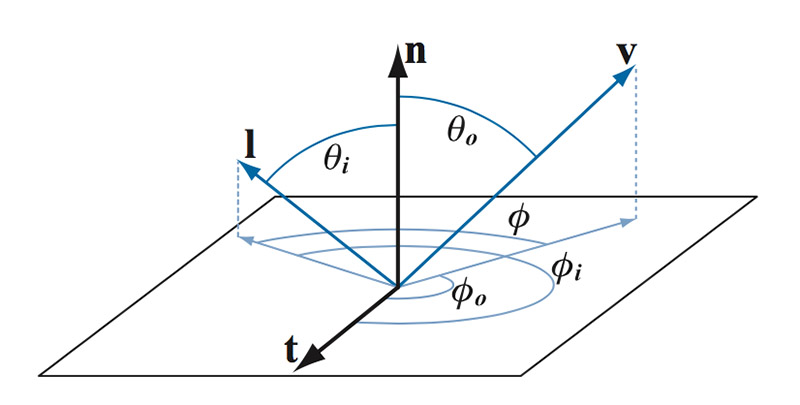Figure 18：BRDF 依赖于射入和射出方向，可以使用四个角来参数化表示，如果是各项同性的话三个角表示。$n$ 是表面的法线，$l$ 是入射光方向向量，$v$ 是射出方向向量，$t$ 是切线向量（只有在各向异性中使用，当 $l$ 和 $v$ 绕着 $n$ 旋转时，反射行为发生变化）。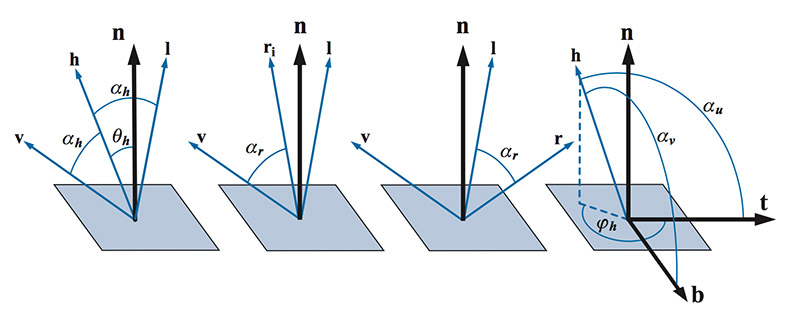Figure 19: 在 BRDF 计算中，常用到的一些角度。

BRDF 有两种直观的解释。第一种解释是，给定一个从某个角度的入射光线，BRDF 给出表面上所有射出方向上的反射和散射光的相对分布（Figure 20 右图）。第二种解释是，给定观察方向，BRDF 给出每一个射入方向上的对射出光线的相对贡献（Figure 20 左图）。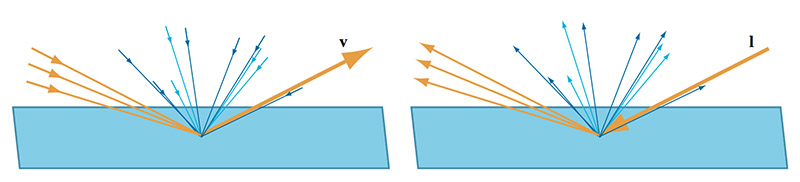Figure 20: 右图为第一种解释。左图为第二种解释。

BRDF 是一个光谱量。理论上输入和输出的波长需要额外的参数。然而，实际情况中，不同的波长之间是独立的，不会产生相互作用，每一个射出光的波长只受相同波长的射入光的影响。这就意味着我们使用更简单的光颜色来代替波长。

$L_o(v) = \int_\Omega f(l,v) \otimes L_i(l)(n \cdot l) d\omega_i$

$f(l,v) = f(v,l)$

$\forall l, \int_\Omega f(l,v)(n \cdot l) d\omega_o \leq 1$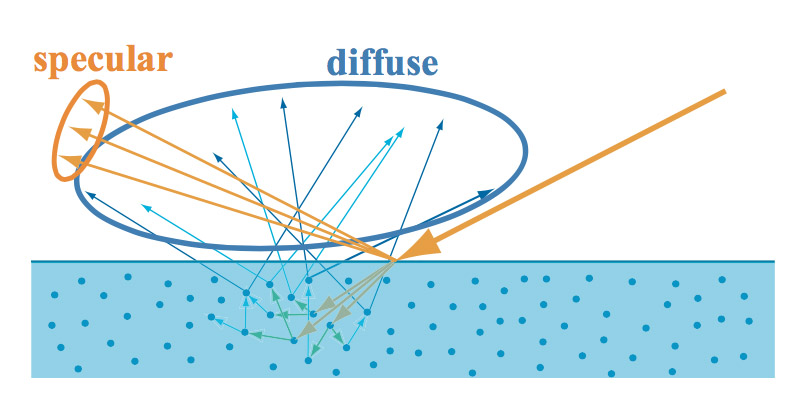Figure 21: BRDF 镜面反射项用来描述表面反射（黄色），BRDF 漫反射项描述次表面散射（蓝色）。

### 表面反射（镜面反射项）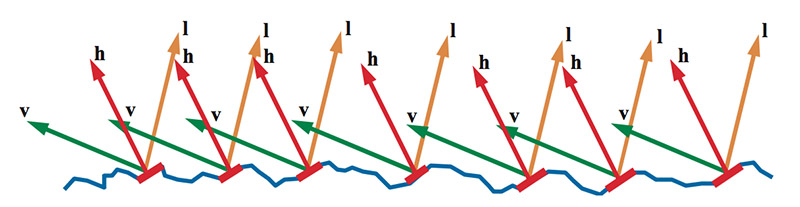Figure 22: $m=h$ 的微表面使得 $l$ 反射到 $v$。其它微表面没有对 BRDF 产生贡献。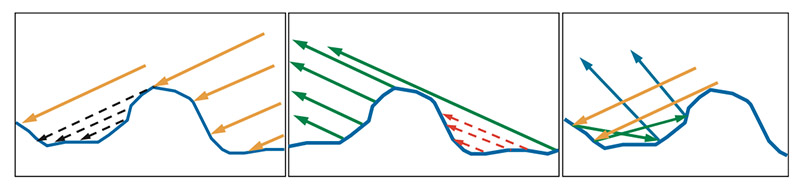Figure 23：左图中，我们看到 $l$ 方向的一部分光被遮挡住了，所以部分微表面在阴影中，无法接受到光（当然也无法反射光）。中间图，一些微表面对于观察者来说是不可见的，所以这些微表面反射的光是不会被看到的。这两种情况，微表面都不会对 BRDF 产生贡献。在实际情况中（右图），这些光并不会简单的消失，而是在微表面中相互反射，最终一部分会被反射到观察方向上。微表面理论忽略这种内部间的反射。

$f_{ufacet}(l,v) = { F(l,h)G(l,v,h)D(h) \over 4(n \cdot l)(n \cdot v) }$

### Fresnel 反射项

Fresnel 反射项计算光从光学平面反射的比例。这个值依赖于两件事：入射角（入射光线和法线的夹角）和材质的反射率。可见光谱中的发射率各不相同，为了制作上的方便，Fresnel 反射是一个光谱量（RGB）。我们同时知道，RGB 的每一个分量都在 0 到 1 之间，因为表面无法反射小鱼 0% 或者多余 100% 的入射光。而且我们只关心那些活动的微表面（$m=h$），产生 Fresnel 反射的角度是在 $l$ 和 $h$ 之间。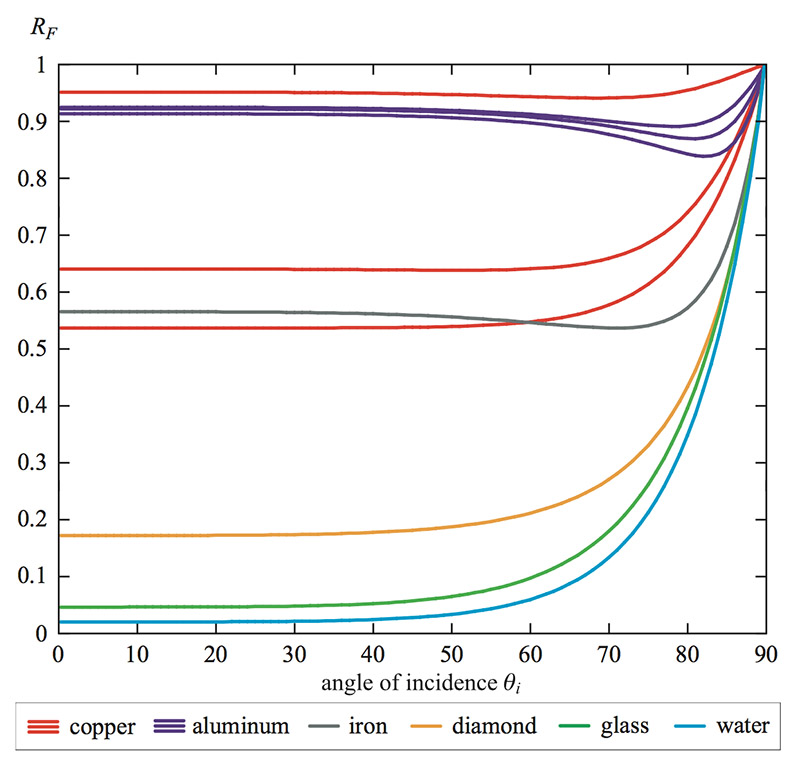Figure 24:不同物质的不同的 Fresnel 反射。铜和铝在可见光谱上的反射率是有很大不同的，上图中反射表现为三调分开的曲线分别表示 RGB。铜的 R 曲线是最高的，然后是 G，最后是 B（因此总体是偏红的）。铝的 B 曲线是最高的，然后是 G，最后是 R。

$c_{spec}$ 看起来是近似模拟 Fresnel 反射的一个理想的参数。并且 Schlik 也给出了一个高效且较为精确近似的方法：

$F_{Schlic} (c_{spec},l,n) = c_{spec} + (1 - c_{spec})(1 - (l \cdot n))^5$

$F_{Schlic} (c_{spec},l,n) = c_{spec} + (1 - c_{spec})(1 - (l \cdot h))^5$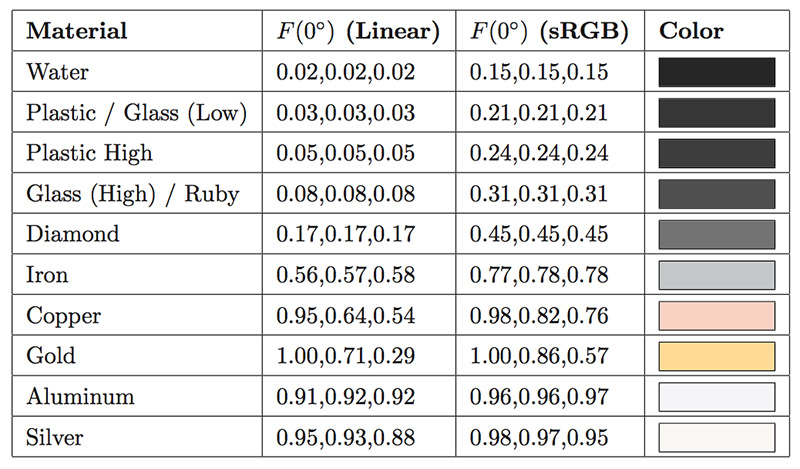Table 1：各种材质当 $F(0^o)$ 时的值。

### 法线分布项

$D()$ 决定了镜面高光的尺寸、亮度以及形状。在图形学资料中有几种不同的法线分布函数，所有的都是类高斯（Gaussian）的方法，使用了类似粗糙度和其它一些参数（各项异性通常会有两个不同的参数）。当粗糙度减小的时候，微表面的法线 $m$ 会更聚集到宏观表面法线周围，$D()$ 的值会变得很高（在极限情况下，完美的镜面时，$m=n$ 时，值为无穷大）。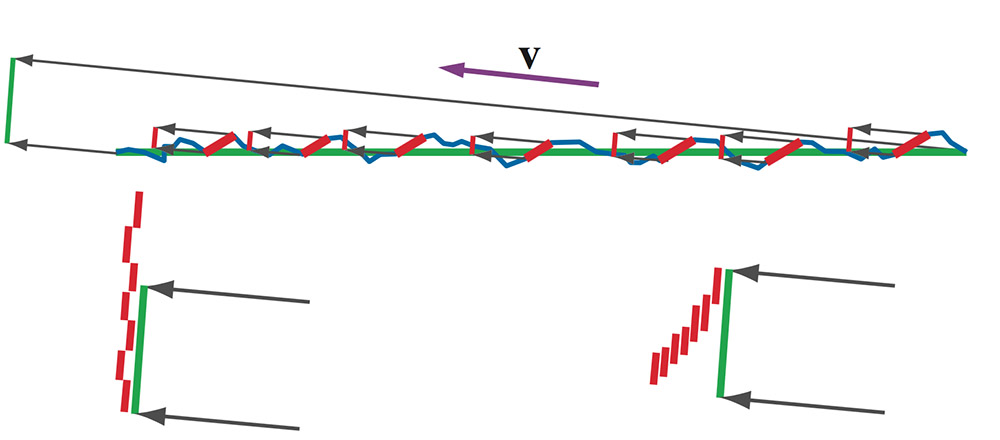Figure 25: 上图中，宏观表面用绿色标记，蓝色的表示凹凸不平的微表面，红色表示活动的微表面。宏观表面区域在观察方向上的的投影在左上角绿色线段表示。每个活动微表面的投影区域用分开的红色线段表示。左下图中，红色微表面区域相加但是并没有考虑遮挡，其结果是活动区域大于总区域。这是不合理的，BRDF 反射的能量比接受到的还要多。右下图中，可以看到使用一种考虑了遮挡的方式。叠加的部分不再被多次计算，活动区域小于总的区域。当观察角度更低的时候，这种对比就更明显了，忽略遮挡将导致 BRDF 反射的能量上千倍于接受的能量（当观察角度无限接近 $90^o$ 时，反射能量会变得无穷大）。

### 次表面散射（漫反射项）

$f_{Lambert}(l,v) = {c_{diff} \over \pi}$

$c_{diff}$ 是漫反射光照的一部分。就像 $c_{spec}$ 一样，这是一个使用 RGB 分量表示的值，并且限制在 0 到 1 之间，相当于大多数人认为的表面色。这个参数通常是指漫反射颜色。

Non-Lambertian 漫反射项试图模型化在掠射角（glancing angles）时镜面反射项和漫反射项的权衡，以及当规模大于散射距离时的表面粗糙度效果。

## 实现着色

### 精确光源（Punctual Light Sources）

$\forall l \mid \angle (l,l_c) > \varepsilon, L_{tiny}(l) = 0 \\ if \ l_c = n, then \ c_{light} = {1 \over \pi} \int_\Omega L_{tiny}(l) (n \cdot l) d\omega_i$

$if \ l_c = n, then \ c_{light} = \lim_{\varepsilon \to 0} \left( {1 \over \pi} \int_\Omega L_{tiny}(l) (n \cdot l) d\omega_i \right)$

$c_{light} = \lim_{\varepsilon \to 0} \left( {1 \over \pi} \int_\Omega L_{tiny}(l) d\omega_i \right)$

$\lim_{\varepsilon \to 0} \left( \int_\Omega L_{tiny}(l) d\omega_i \right) = \pi c_{light}$

$L_o(v) = \lim_{\varepsilon \to 0} \left( \int_\Omega f(l,v) \otimes L_{tiny}(l) (n \cdot l) d\omega_i \right) = f(l_c,v) \otimes \lim_{\varepsilon \to 0} \left( \int_\Omega L_{tiny}(l) d\omega_i \right) (n \cdot l_c)$

$L_o(v) = \pi f(l_c,v) \otimes c_{light} \underline{ (n \cdot l_c) }$